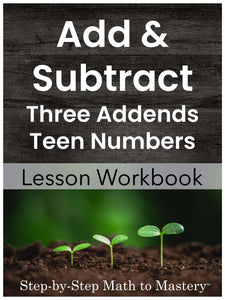• \$12.00
Unit price per
Shipping calculated at checkout.

### 42 Step-by-Step Math Lessons | Special Education Math Intervention | First Grade Math Level

This Three Addend Addition and Addition & Subtraction to 20 lesson workbook includes an example IEP goal, 42 step-by-step math lessons, reviews and assessments. This evidence-based math intervention is tied to first grade standards and is great for special education math goals and tier 2 small group math interventions (RTI).

Three Addends:  This section of the workbook contains lessons on how to add three numbers with sums to 20. Students learn to say the biggest number and count up on the smaller numbers using touch dots.  Adding three numbers is a pre-skill for adding multi-digit numbers with carrying.  These lessons can help prepare your students for adding larger numbers in the future.

Teen Numbers Plus or Minus One-Digit Numbers:  These lessons are a continuation of Adding and Subtracting Numbers to 10.  Students use the same touch dot patterns and follow the same steps:  Say the big number, tap the dots on the smaller number, and count up/back.

These sequential lessons are easy to teach, with enough material for 9 weeks of instruction!

42 Lessons
15 Reviews
7 Tests

134 Pages

9 Weeks of Instruction

## Students Will Be Able To:

• Add to solve horizontal and vertical equations without touch dots.
• Listen to or read word problems and set up a three-addend equation to solve.
• Add teen numbers to one-digit numbers using the count on strategy (with touch dots)
• Add twenty plus one-digit numbers using the count on strategy
• Subtract numbers 1-9 using the touch dot strategy.
• Fade from drawing the touch dots on the smaller number to pretending they are there and tapping while counting back.

## Teacher Feedback:

❤️ "As a special education teacher I have found all of the math resources I've used from this seller to be outstanding. I have been a sped teacher for 15 years, and have struggled with finding the best way to break down math into step by step, easy to follow and understand lessons. I found these math resources and they all provide example IEP goals, guided practice and independent work, anchor charts, visuals, and explicit, sequential, & structured lessons for my students. I appreciate the time and effort put into these products, and the fair prices! Thank you!" -Amber M.

❤️ "This was great! Very little prep but very effective." -Mickelle B.

❤️ "I had good results with my special education math groups with this." -Julia P.

❤️ "These worksheets are the perfect amount of problems for my intervention groups. They allow for practice and fluency, without being repetitive." -Juliet B.

## Lessons Are Tied to These Standards:

Solve word problems that call for addition of three whole numbers whose sum is less than or equal to 20, e.g., by using objects, drawings, and equations with a symbol for the unknown number to represent the problem.

Relate counting to addition and subtraction. (e.g., by counting on 2 to add 2)

Add and subtract within 20, demonstrating fluency for addition and subtraction within 10.  Use strategies such as counting on; making ten; decomposing a number leading to a ten; using the relationship between addition and subtraction; and creating equivalent but easier or known sums.

Fluently add and subtract within 20 using mental strategies.  By end of Grade 2, know from memory all sums of two one-digit numbers.

### Not sure where to start?

Watch a video overview of Step-by-Step Math to Mastery or read the transcript here.

### Step-by-Step Math to Mastery™ Resources:

Make math easier to understand.

• Help students over math hurdles with clear, sequential, scaffolded lessons.
• Prevent overwhelm. Build student confidence.

Make math easier to teach.

• Save hours of planning and piecing together materials.
• Paraeducators can deliver quality instruction independently. Open & teach.

### Reasons You'll Love Step-by-Step Math to Mastery™ Resources

• Boosts student confidence and progress
• Time-saving: print and teach
• Easy prep: only black ink is needed
• Easy to teach, paraeducator-friendly
• Example IEP goals and shorter term objectives
• Consistent & predictable format
• Lots of practice repetitions
• Scaffolded with structured workspaces
• Fewer problems on a page, white space, minimal visual clutter
• Tied to standards
• Explicit/Direct instruction
• Systematic & sequential
• Mastery approach--teaching one topic at a time, one strategy at a time
• Lessons have "I Can" statements, model problems, guided practice, & independent practice
• Each workbook can be used individually as a stand-alone intervention for that skill or they can be used together, taking students from the basics of number sense and addition in first grade all the way to dividing fractions and decimals in fifth grade.
• Can also be used with older students (middle & high school) to help fill the gaps in their learning.